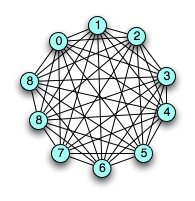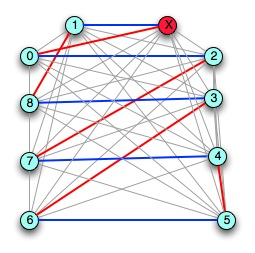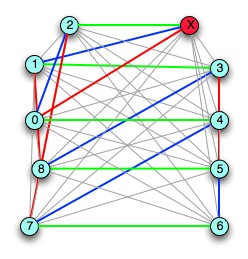# Edge Coloring and Graph Turning

In addition to doing vertex and face colorings of a graph, you can also do edge colorings. In an edge coloring, no two edges which are incident on the same vertex can share the same color. In general, edge coloring doesn’t get as much attention as vertex coloring or face coloring, but it can be an interesting subject. Today I’m going to show you an example of a really clever visual proof technique called *graph turning* to prove a statement about the edge colorings of complete graphs.
Just like a graph has a chromatic index for its vertex coloring, it’s got a chromatic
index for its edge coloring. The edge chromatic index of a graph G is the minimum number of colors in any edge-coloring of G. The theorem that I’m going to prove for you is about the edge chromatic index of complete graphs with 2n vertices for some integer n:
**The edge-chromatic index of a complete graph K2n = 2n-1.**

To start with, we’ll set an *lower bound* on the value of the edge-chromatic index, which is simple. The edge chromatic index of a graph is greater than or equal to the highest
degree of any vertex in that graph. The reason why is obvious by definition: the edge coloring must have distinct colors for any edges that are incident on the same vertex – so any coloring wit less colors than the degree of some vertex *must* necessarily have two edges of that vertex with the same color.
In fact, we can be even stronger than that for regular graphs. For a n-regular graph (that is, a graph where every vertex has *n* edges), the edge-chromatic index must be either n or n+1.
Every vertex in K2n is degree 2n-1. So to prove the theorem, all we need to do is show that there is an edge coloring with degree 2n-1.The way that we’ll do that is using a technique called graph turning. Take the graph, and pick one vertex. Call it vertex *X*, which we’ll use as the *pivot* of the turning. Take the other vertices, and number them from 0 to 2n-2. (As a running example down the right, we’ll use K10 – K2n for n=5, which must have a coloring with 9 colors.)
To do the turning, you set up the graph as follows:* Drag the pivot out of the graph. Put vertex 0 to the left of it. Then in two columns under it, put 1, 2, 3, … on the right side under the pivot, and 2n-2, 2n-3, …. on the left, with the two columns
lining up in rows. (See the example to the side.) This two column structure, with
X at the top of the right-hand column, is what we’ll use for turning.
* Take this initial configuration, with X and 0 at the top. Each horizontal edge is
going to get color 0. So 0→x, 2n-2→1, 2n-3→2, and so on, get color 0.
(In our example, it’s (0,x), (8,1), (7,2), (6,3), (5,4)).)* Now we’re going to *turn* the graph. Keep the pivot where it is, but rotate all of the
other vertices counterclockwise – so now 1 is paired with X, 0 is lined up with 2, 2n-2
is lined up with with 3, and so on. Now, all of the edges that are horizontal get color 1.* Turn again: X gets paired with 2, 1 with 3, 0 with 4, 2n-2 with 5; again, the horizontals get the next color, color 2.
Each turning of the graph will select a new set of N edges to be colored. How many times can we turn it? Each node 0 through 2n-1 (that is, 2n+1 nodes) will get paired with X – there are 2n-2 turns, assigning 2n-1 colors (the initial color, plus one for each turning). If you look at the example diagrams, you can see that the colored edges can’t get to the horizontal where they’d be colored in less that 2n-1 turns. So this produces a coloring with 2n-1 colors. Since we already know that the edge-coloring has *at least* 2n-1 colors, we’ve proved that the chromatic index is exactly 2n-1, because it can be colored in that minimum.
Then, as a simple corollary, we can show that a complete graph with 2n-1 vertices *also* has edge chromatic index 2n-1. It’s really easy. There are two parts to the proof: first, showing that the graph *can* be edge-colored in 2n-1 colors; and then showing that it *can’t* be edge-colored in 2n-2 colors.
To show that it can be edge-colored in 2n-1 colors, we can just add another vertex, turning it into K2n, coloring it as above, and then removing the extra vertex, keeping the colors of the edges.
To show that it *can’t* be edge-colored in 2n-2 colors, there’s a simple combinatorial trick we can use:
* The complete graph with 2n-1 vertices has ((2n-1) choose 2) edges, or (2n-1)(n-1)=2n2-3n+1 edges.
* Suppose that we *could* color all of the edges with 2n-2 colors. How many edges would
there be with each color? The average would be (2n-1)(n-1)/2n-2 – the total number of edges divided by the number of colors.
* If the coloring works, than there can’t be more that N-1 edges with the same color,
or else there’d be a vertex with the same color for two of its edges: since the number
of edges is (2n-1)(n-1), and there are 2n-1 vertices, then if any color is assigned to
more than n-1 edges, then some vertex must have two edges with the same color.
* But since the number of edges is (2n-1)(n-1), and the number of colors 2N-2 is less that 2n-1, then the average number of edges per color must be *greater than* n-1, which means that at least one color is assigned to n edges.
Since that’s a contradiction, we know that we can’t color K2n-1 in less than 2n-1 colors.

## 0 thoughts on “Edge Coloring and Graph Turning”

1.Jonathan Vos Post

arXiv:0707.1177
Title: A unit distance graph with ambiguous chromatic number

Authors: M. S. Payne
Comments: 4 pages. Submitted to Graphs and Combinatorics
Subjects: Combinatorics (math.CO)
Saharon Shelah and Alexander Soifer recently presented some examples of infinite graphs whose chromatic numbers depend on the axioms chosen for set theory. The existence of such graphs may be relevant to the famous Chromatic Number of the Plane problem. In this paper a new example of a graph with ambiguous chromatic number is presented. This graph is a unit distance graph in the plane and hence may be seen as further evidence that the chromatic number of the plane might depend on set theory.